>  Test: BITSAT Mock Test- 3

# Test: BITSAT Mock Test- 3

Test Description

## 150 Questions MCQ Test BITSAT Mock Tests Series & Past Year Papers | Test: BITSAT Mock Test- 3

 1 Crore+ students have signed up on EduRev. Have you?
Test: BITSAT Mock Test- 3 - Question 1

### An electric motor operates at 20 r.p.s. What will be the approximate power delivered by the motor, if it supplies a torque of 75 N-m?

Test: BITSAT Mock Test- 3 - Question 2

### Which of th following transitions in a hydrogen atom emits the photon of highest frequency?

Test: BITSAT Mock Test- 3 - Question 3

### The ratio of the longest to shortest wavelengths in Brackett series of hydrogen spectra is

Detailed Solution for Test: BITSAT Mock Test- 3 - Question 3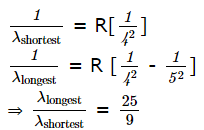Test: BITSAT Mock Test- 3 - Question 4

Potential difference across the terminals of a battery is 50 V, when 11 A current is drawn. And it is 60 V, when 1 A current is drawn. The e.m.f. and internal resistance of battery is

Detailed Solution for Test: BITSAT Mock Test- 3 - Question 4

e = v + Ir
e = emf
V = potential difference
r = internal resistance
Here e = 50 + 11r .....(1)
and e = 60 + 1r .....(2)
Solving these equations (1) and (2) for e and r we get
e = 61V and r = 1 Ω

Test: BITSAT Mock Test- 3 - Question 5

The unit of specific conductivity is

Test: BITSAT Mock Test- 3 - Question 6

When an inert gas is filled in the place vacuum in a photo cell, then

Detailed Solution for Test: BITSAT Mock Test- 3 - Question 6 In the presence of inert gas photoelectrons emitted by cathode ionise the gas by collision and hence the current increases.
Test: BITSAT Mock Test- 3 - Question 7

Light of frequency 4v₀ is incident on the metal of the threshold frequency v₀. The maximum kinetic energy of the emitted photoelectrons is

Test: BITSAT Mock Test- 3 - Question 8

An L.C. circuit contains 10 mH inductor and a 25 μ F capacitor. The resistance of the circuit is negligible. The energy stored in the circuit is completely magnetic at time (in milli seconds) being measured from the instant when the circuit is closed

Detailed Solution for Test: BITSAT Mock Test- 3 - Question 8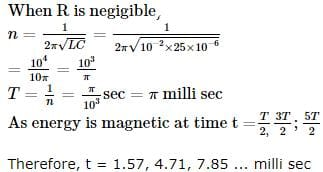Test: BITSAT Mock Test- 3 - Question 9

Minimum no. of 8 μF and 250 V capacitors, used to make a combination of 16 μF and 1000 V, is

Detailed Solution for Test: BITSAT Mock Test- 3 - Question 9

To get 1000 volts from 250 volts, we have to connect Four capacitors in series.

For such a series connection, effective capacitance is  2 micro farad, because 1/Ceffective = 1/C1 + 1/C2 +1/C3 +1/C4 = 1/8 + 1/8 + 1/8 + 1/8 = 1/2.
Therefore Ceffective  = 2 microfarad. Hence the series combination is equivalent of 2 microfarads, 1000 Volts.

Now we have to connect 8 of these four capacitor units in parallel to get 16 micro farads capacitor because in parallel combination, effective capacitance is equal to summation of each capacitor.  Hence capacitors required will be:

4 numbers for each series combinations * 8 such combinations = 32 capacitors.

Test: BITSAT Mock Test- 3 - Question 10

The ratio of charge to potential of a capacitor is known as its

Test: BITSAT Mock Test- 3 - Question 11

To aviod slipping while walking on ice, one should take smaller steps because of the

Test: BITSAT Mock Test- 3 - Question 12

The acceleration due to gravity on the planet A is 9 times the acceleration due to gravity on planet B. A man jumps to a height of 2 m on the surface of A. What will be the height of jump by the same person on the planet B?

Test: BITSAT Mock Test- 3 - Question 13

When a body is heated, which colour corresponds to highest temperature

Test: BITSAT Mock Test- 3 - Question 14

A vessel contains a mixture of one mole of oxygen and two moles of nitrogen at 300 K. The ratio of the average rotational kinetic energy per O₂ molecule to that per N₂ molecule is

Test: BITSAT Mock Test- 3 - Question 15

When a charged particle moves perpendicular to a magnetic field, then

Test: BITSAT Mock Test- 3 - Question 16

The force between two short bar magnets with magnetic moments M₁ and M₂ whose centres are r meter apart is 8 N, when their axes are in same line. If the separation is increased to 2 r, the force between them is reduced to

Detailed Solution for Test: BITSAT Mock Test- 3 - Question 16

Explanation : In magnetic dipole, force ∝ 1/r4

Hence, new force = 8/24

= 8/16

= 0.5N

Test: BITSAT Mock Test- 3 - Question 17

Oil spreads over the surface of water, whereas water does not spread over the surface of oil, because

Test: BITSAT Mock Test- 3 - Question 18

A 20 cm long capillary tube is dipped in water. The water rises up to 8 cm. If entire arrangement is put in a freely elevator, the length of water column in the capillary tube will be

Test: BITSAT Mock Test- 3 - Question 19

The breaking stress of a wire depends upon

Test: BITSAT Mock Test- 3 - Question 20

A particle covers 150 m in 8th second when starts from rest, its acceleration is

Test: BITSAT Mock Test- 3 - Question 21

The resultant of two forces, one double the other in magnitude, is perpendicular to the smaller of the two forces. The angle between the two forces is

Test: BITSAT Mock Test- 3 - Question 22

Two vectors A̅ and B̅ are such that A̅ + B̅ = A̅ - B̅. Then

Detailed Solution for Test: BITSAT Mock Test- 3 - Question 22

Explanation : A + B = A - B

cancelling A both sides, we get

B = - B

Taking -B to other side, we get

2B = 0

B = 0

Test: BITSAT Mock Test- 3 - Question 23

A car of mass 12kg at rest explodes into two pieces of masses 4kg and 8kg which move in opposite direction. If the velocity of 8kg piece is 6 m/s, the kinetic energy of other piece is

Test: BITSAT Mock Test- 3 - Question 24

The image formed by an objective lens of a compound microscope is

Test: BITSAT Mock Test- 3 - Question 25

A chimpanzee, swinging on a swing in a sitting position, stands up suddenly. The time period will

Test: BITSAT Mock Test- 3 - Question 26

An instantaneous displacement of a simple harmonic oscillator is x = A cos (ωt + π/4 ). Its speed will be maximum at time

Detailed Solution for Test: BITSAT Mock Test- 3 - Question 26

The velocity is the time derivative of displacement: v = dy/dt = -Aω (sinωt + π/4 ).
Its maximum magnitude equal to Aω is obtained when ωt = π/4 , from which t = π/4ω

Test: BITSAT Mock Test- 3 - Question 27

A ray of light is incident on a plane mirror at an angle of 60o . The angle of deviation produced by the mirror is

Test: BITSAT Mock Test- 3 - Question 28

Two spheres of masses 2M and M are initially at rest at a distance R apart. Due to mutual force of attraction, they approach each other. When they are at separation R/2, the acceleration of the centre of mass of spheres would be

Test: BITSAT Mock Test- 3 - Question 29

A soild sphere, disc and solid cylinder all of the same mass and made of the same material are allowed to roll down (from rest) on an inclined plane, then

Test: BITSAT Mock Test- 3 - Question 30

A small steel sphere is tied to a string and is whirled in a horizontal circle with a uniform angular velocity ω₁. The string is suddenly pulled so that radius of the circle is halved. If ω₂ is new angular velocity. then

Test: BITSAT Mock Test- 3 - Question 31

If three bulbs 60W, 100W and 200W are connected in parallel, then

Detailed Solution for Test: BITSAT Mock Test- 3 - Question 31

Bulb of higher voltage will glow more

Test: BITSAT Mock Test- 3 - Question 32

A gas performs no work, when it expands

Test: BITSAT Mock Test- 3 - Question 33

A Carnot's engine has an efficiency of 50% at sink temperature 50o C . Calculate the temperature of source.

Detailed Solution for Test: BITSAT Mock Test- 3 - Question 33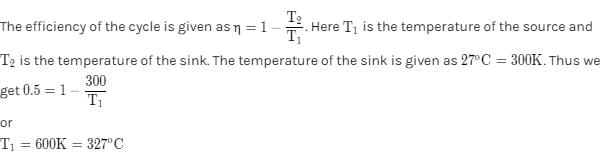Test: BITSAT Mock Test- 3 - Question 34

The dimensions of power are

Test: BITSAT Mock Test- 3 - Question 35

Wave optics is based on wave theory of light put forward by Huygen and modified later by

Test: BITSAT Mock Test- 3 - Question 36

When two coherent monochromatic light beams of intensities I and 4I are superimposed, the ratio between maximum and minimum intensities in the resultant beam is

Test: BITSAT Mock Test- 3 - Question 37

In the stationary waves the phase difference between two particles of the medium situated on the opposite sides of a node is

Test: BITSAT Mock Test- 3 - Question 38

The equation of progressive wave is y = a sin(200t - x). whre x is in meter and t is in second. The velcoity of wave is

Detailed Solution for Test: BITSAT Mock Test- 3 - Question 38

Comparing the given equation with y = a sin (wt - kx) (w = 200, k = 1)
So, v = w/k = 200 m/s

Test: BITSAT Mock Test- 3 - Question 39

A force of 170 N is applied to a body of mass 5 kg which raised it vertically to a height of 10 m. The velocity of the body at this height will be

Test: BITSAT Mock Test- 3 - Question 40

Two equal masses are attached to the ends of a spring of spring constant k. The two masses are pulled out equally to stretch the spring by 'x'. The work done by the spring on each mass is

Test: BITSAT Mock Test- 3 - Question 41

Reaction of phenol with chloroform and sodium hydroxide to give o-hydroxybenzaldehyde involves the formation of

Test: BITSAT Mock Test- 3 - Question 42

Which of the following reagent react differently with HCHO and CH₃CHO and CH₃COCH₃

Test: BITSAT Mock Test- 3 - Question 43

The orientation of an atomic orbital is governed by

Test: BITSAT Mock Test- 3 - Question 44

For principle quantum number n = 4, the total number of orbitals having l = 3 is

Test: BITSAT Mock Test- 3 - Question 45

The random errors can be eliminated by

Test: BITSAT Mock Test- 3 - Question 46

1.5 mol of O₂ combine with Mg to form oxide MgO. The mass of Mg (at. mass 24) that has combined is

Test: BITSAT Mock Test- 3 - Question 47

Which of the following is the strongest nucleophile?

Detailed Solution for Test: BITSAT Mock Test- 3 - Question 47

A negatively charged nucleophile is always stronger than a neutral one

Test: BITSAT Mock Test- 3 - Question 48

Which of the following does not show electromeric effect?

Test: BITSAT Mock Test- 3 - Question 49

Which carbohydrate is an essential constituent of plant cells?

Test: BITSAT Mock Test- 3 - Question 50

Cane sugar on hydrolysis gives

Test: BITSAT Mock Test- 3 - Question 51

Dimerisation in carboxylic acid is due to

Test: BITSAT Mock Test- 3 - Question 52

The maximum number of hydrogen bonds formed by a water molecule in ice, is

Test: BITSAT Mock Test- 3 - Question 53

A well stoppered thermos flask contains some ice cubes . This is an example of

Test: BITSAT Mock Test- 3 - Question 54

Just before attaining equilibrium by a reversible reaction, it is found that

Test: BITSAT Mock Test- 3 - Question 55

The rate constant is given by the equation K = P Ze(-E/RT). Which factor should register a decrease for the reaction to proceed more rapidly?

Test: BITSAT Mock Test- 3 - Question 56

Which one of the following relations is correct with respect to first (I) and second (II) ionization potential of Sodium and Magnesium ?

Detailed Solution for Test: BITSAT Mock Test- 3 - Question 56

As we move across a period from left to right, the ionisation potential increases with increase in atomic number. Thus, the first ionisation energy of Mg is higher than Mg, i.e.
INa < IMg.
But after removing one electron Na gets inert gas electronic configuration (1s2 2s2 2p6) , which is more stable. Hence, the second ionization potential of Na is higher than Mg, i.e.
IINa > IIMg .

Test: BITSAT Mock Test- 3 - Question 57

What is the amount of heat liberated (in k.cal) when one mole of aquesous NaOH reacted with one mole of aqueous HCl solution?

Test: BITSAT Mock Test- 3 - Question 58

Azo dyes are prepared from

Detailed Solution for Test: BITSAT Mock Test- 3 - Question 58

Correct Option is D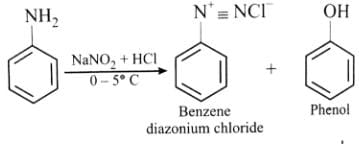Test: BITSAT Mock Test- 3 - Question 59

The no. of unpaired electrons in the complex ion [CoF₆]3⁻ is (Atomic no. Co = 27)

Test: BITSAT Mock Test- 3 - Question 60

Which of the following has the largest atomic radius?

Test: BITSAT Mock Test- 3 - Question 61

Which of the following is formed when K₂Cr₂O₇ reacts with concentrated H₂SO₄ in cold?

Test: BITSAT Mock Test- 3 - Question 62

The unit of electrochemical equivalent is

Test: BITSAT Mock Test- 3 - Question 63

Among the following compounds (I-III), the correct order in reaction with electrophile is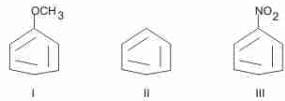Test: BITSAT Mock Test- 3 - Question 64

Increasing order of stability among the three main conformations (i.e.,Eclipse ,Anti,Gauche) of 2-fluoroethanol is

Test: BITSAT Mock Test- 3 - Question 65

Water can be tested by

Test: BITSAT Mock Test- 3 - Question 66

The number of moles of acid or base required by 1 litre of buffer to alter its pH by one unit is called

Test: BITSAT Mock Test- 3 - Question 67

The isomer which bears object-image relationship but do not superimpose on each other is called

Test: BITSAT Mock Test- 3 - Question 68

How many monochlorobutanes will be possible on chlorination of n-butane?

Test: BITSAT Mock Test- 3 - Question 69

Oxalic acid reacts with concentrated H₂SO₄ to give a mixture of two gases. When this mixture is passed through caustic potash, one of the gases is absorbed. What is the product formed by the absorbed gas with caustic potash?

Test: BITSAT Mock Test- 3 - Question 70

Oxidation state of chlorine in hypochlorous acid is

Test: BITSAT Mock Test- 3 - Question 71

Which of the following does not give a borax bead test?

Test: BITSAT Mock Test- 3 - Question 72

Which of the following element is prepared by electrolysis

Test: BITSAT Mock Test- 3 - Question 73

Laughing gas is

Test: BITSAT Mock Test- 3 - Question 74

Which of the following is not a synthetic polymer?

Test: BITSAT Mock Test- 3 - Question 75

Which of the following compounds is used in gun powder?

Test: BITSAT Mock Test- 3 - Question 76

Excess of Na⁺ ions is human system causes

Test: BITSAT Mock Test- 3 - Question 77

Which of the following set of variables give a straight line with a negative slope when plotted? (P = Vapour pressure; T = Temperature in K)

Test: BITSAT Mock Test- 3 - Question 78

A bottle of cold drink has 200 ml liquid in which CO₂ is 0.1 molar. If CO₂ behaves as ideal gas the volume of CO₂ at S.T.P. solution of cold drink is

Test: BITSAT Mock Test- 3 - Question 79

The formula of metal chloride is MCl3. It contains 20% of metal. The atomic weight of metal is approximately

Test: BITSAT Mock Test- 3 - Question 80

Gold number is minimum in case of

Test: BITSAT Mock Test- 3 - Question 81

The area bounded by the curve y = sec x, the x-axis and the lines x = 0 and x = π/4 is

Test: BITSAT Mock Test- 3 - Question 82

In the expansion of ((x4) - (1/x3))15, the coefficient of x39 is

Test: BITSAT Mock Test- 3 - Question 83

If the line ax + by + c = - touches the circle x2 + y2 - 2x = 3 and is normal to the circle x2 + y2 + 2x - 4y + 1 = 0, then (a,b) are respectively

Test: BITSAT Mock Test- 3 - Question 84

Area of the curve x2+y2=2ax is

Test: BITSAT Mock Test- 3 - Question 85

If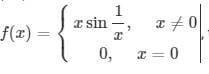then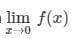is equal to

Test: BITSAT Mock Test- 3 - Question 86

The differential equation | d y d x | + | y | + 3 = 0 admits

Test: BITSAT Mock Test- 3 - Question 87

Differential equation, for y = A cosαx + B sinαx where A and B are arbitrary constants is

Detailed Solution for Test: BITSAT Mock Test- 3 - Question 87

y = A Cosαx + B Sinαx
dy dx = -α A Sinαx + αB Cosαx
d 2 y dx 2 = - α2A Cosαx - α2B Sinαx
d 2 y dx 2 = - α2 (A Cosαx + B Sinαx)
d 2 y dx 2 = - α2y
d 2 y dx 2 + α2y = 0

Test: BITSAT Mock Test- 3 - Question 88

The solution of 2xydydx = 1 + y2 is

Detailed Solution for Test: BITSAT Mock Test- 3 - Question 88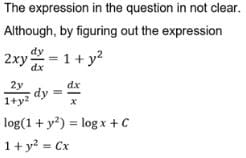Test: BITSAT Mock Test- 3 - Question 89

If f(x)=logx2log(x), then at x=e, f'(x)=

Test: BITSAT Mock Test- 3 - Question 90

If sin (x + y) = log (x + y), then dy/dx =

Detailed Solution for Test: BITSAT Mock Test- 3 - Question 90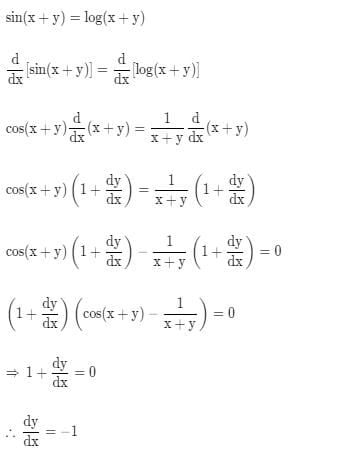Test: BITSAT Mock Test- 3 - Question 91

The sum of the series 2/1! + 4/3! + 6/5! + .....∞ equals

Detailed Solution for Test: BITSAT Mock Test- 3 - Question 91

Explanation : e = ∑(j = 0 to ∞) 1/j!

and group every even term with the next one :

e = ∑(j = 0 to ∞) 1/j! = ∑(k = 0 to ∞) 1/(2k)! + 1/(2k+1)!

= ∑(k = 0 to ∞) [2k+1/(2k+1)! + 1/(2k+1)!]

= ∑(k = 0 to ∞) 2k+2/(2k+1)!.

2n+2/(2n+1)!

= 2n+1/(2n+1)! + 1/(2n+1)! = 1/(2n)! + 1/(2n+1)!

so

∑(n = 0 to ∞) 2n+2/(2n+1)!

= ∑(n = 0 to ∞) 1/(2n)! + 1/(2n+1)!

= cosh1 + sinh1

= e

Test: BITSAT Mock Test- 3 - Question 92

In wind storm a tree has broken and upper portion touches the ground at a distance 10m from the foot of the tree and makes an angle 45owith the ground. Then the total height of the tree is

Test: BITSAT Mock Test- 3 - Question 93

The st. line lx + my + n = 0 touches the hyperbola x2/a2 - y2/b2 = 1 if

Test: BITSAT Mock Test- 3 - Question 94

The parabolas y2= 4x and x2 = 4y divide the square region bounded by the lines x = 4, y = 4 and the coordinate axes. If S₁, S₂, S₃ are respectively the areas of these parts numbered from top to bottom; then S₁ : S₂ : S₃ is

Test: BITSAT Mock Test- 3 - Question 95

The area of the region bounded by the parabola y = x2 + 1 and the straight line x + y = 3 is given by

Test: BITSAT Mock Test- 3 - Question 96

If tan⁻1(2x)+tan⁻1(3x)=(π/4), then x=

Test: BITSAT Mock Test- 3 - Question 97

the value of f(0) so that the function f(x) = (2x - sin⁻1 x)/(2x + tan⁻1x) is continuous at each point on its domain is

Test: BITSAT Mock Test- 3 - Question 98

If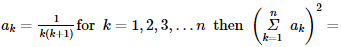Test: BITSAT Mock Test- 3 - Question 99

If A2 - A + I = 0, then the inverse of A is

Test: BITSAT Mock Test- 3 - Question 100

The number of solutions of 2x + y = 4, x - 2 y = 2, 3x + 5y = 6 is

Test: BITSAT Mock Test- 3 - Question 101

The minimum distance from the point (4, 2) to the parabola y2 = 8x is

Test: BITSAT Mock Test- 3 - Question 102

If x=a+b,y=aω+b ω2, z=a ω2 + bω, then xyz equals

Test: BITSAT Mock Test- 3 - Question 103

The angle between lines represented by 4x2-24xy+11y2=0 is

Test: BITSAT Mock Test- 3 - Question 104

The axis of the parabola 9y2-16x-12y-57=0 is

Test: BITSAT Mock Test- 3 - Question 105

If 2 y = 5 x + k is a tangent to the parabola y2 = 6 x then k =

Test: BITSAT Mock Test- 3 - Question 106

All letters of the word EAMCET can be written in all possible ways. In how many ways can the letters of the word EAMCET be arranged so that two vowels are never together.

Detailed Solution for Test: BITSAT Mock Test- 3 - Question 106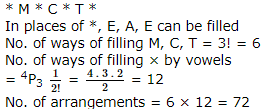Test: BITSAT Mock Test- 3 - Question 107

How many words can be formed from the letters of the word MATHEMATICS?

Test: BITSAT Mock Test- 3 - Question 108

A problem in Statistics is given to three students whose chances of solving it one 1/2, 1/3 and 1/4. The probability that the problem is solved is

Test: BITSAT Mock Test- 3 - Question 109

If A and B are two mutually exclusive events such that P(B) = 2P(A) and A ∪ B = S. Then P(A) is equal to

Test: BITSAT Mock Test- 3 - Question 110

In Δ A B C , If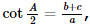, then the triangle is

Test: BITSAT Mock Test- 3 - Question 111

If in a Δ A B C , 3 a = b + c then tan B/2 • tan C/2 is equal to

Detailed Solution for Test: BITSAT Mock Test- 3 - Question 111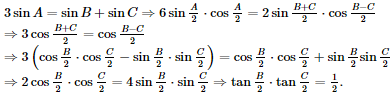Test: BITSAT Mock Test- 3 - Question 112

If both the roots of the quadratic eqution x2 - 2kx + k2 + k - 5 = 0 are less than 5, then k lies in the interval

Test: BITSAT Mock Test- 3 - Question 113

A student read common difference of an A.P. as -2 instead of 2 and got the sum of first 5 terms as -5 Actual sum of first five terms is

Test: BITSAT Mock Test- 3 - Question 114

7th term of an A.P. is 40.Then the sum of first 13 terms is

Test: BITSAT Mock Test- 3 - Question 115

Which of the functions:
f(x) = x2 for all x, g (x) = x2, x ≥ 0 and h (x) = x2, x ≤ 0 have inverse functions :

Test: BITSAT Mock Test- 3 - Question 116

If f (x) = (1)/(1 - x), then f[f{f (x)}] =

Test: BITSAT Mock Test- 3 - Question 117

The equation of a line passing through (3,-4) and perpendicular to line 3x+4y=5 is

Test: BITSAT Mock Test- 3 - Question 118

If at each point of the curve y = x3 - ax2 + x+ 1 the tangent is inclined at an acute angle with the positive direction of the x-axis then

Detailed Solution for Test: BITSAT Mock Test- 3 - Question 118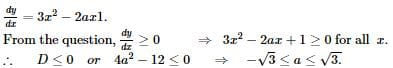Test: BITSAT Mock Test- 3 - Question 119

The centre of the sphere which passes thro' four points (0,0,0),(0,2,0),(1,0,0) and (0,0,4) is

Test: BITSAT Mock Test- 3 - Question 120

The shortest distance between the lines (x - 3)/3 = (y - 8)/-1 = (z - 3)/1 and (x + 3)/-3 = (y + 7)/2 = (z -6)/4 is

Test: BITSAT Mock Test- 3 - Question 121

The set of values of x for which [(tan3x-tan2x)/(1+tan3xtan2x)]=1, is

Test: BITSAT Mock Test- 3 - Question 122

If 0≤x≤π and 81sin2x + 81 cos2x = 30, then x is equal to

Test: BITSAT Mock Test- 3 - Question 123

If cosA=(3/4) then 32 sin(A/2) cos(5A/2)=

Test: BITSAT Mock Test- 3 - Question 124

If θ is the angle between a and b, then a.b≥0 if

Test: BITSAT Mock Test- 3 - Question 125

Vector 2i+aj+k is perpendicular to vector 2i-j-k if a=

Test: BITSAT Mock Test- 3 - Question 126

Find the Antonym of the word occurring in the sentence in capital letter as per the context.
Because of the failure of the monsoon, there was PAUCITY of foodgrains.

Detailed Solution for Test: BITSAT Mock Test- 3 - Question 126

Meaning of Paucity
Smallness of number; fewness
Scarcity; dearth
Synonyms : Scarcity, lack, shortage, rarity, insufficiency
Antonyms : abundance, affluence, plenty

Test: BITSAT Mock Test- 3 - Question 127

Find the Antonym of the word occurring in the sentence in capital letter as per the context.
After swallowing the frog, the snake had become LETHARGIC.

Detailed Solution for Test: BITSAT Mock Test- 3 - Question 127

'Lethargic' means not having any energy.
So its antonym will be Active

Test: BITSAT Mock Test- 3 - Question 128

Fill in the blank with appropriate word.
He finds it difficult to......between blue and green as he is colour blind.

Test: BITSAT Mock Test- 3 - Question 129

Fill in the blank with appropriate word.
Hardly had he arrived .......... it started raining.

Test: BITSAT Mock Test- 3 - Question 130

Choose the one which can be substituted for the given words/sentences
A place where bees are kept is called

Test: BITSAT Mock Test- 3 - Question 131

Choose the one which can be substituted for the given words/sentences :
Code of dipomatic etiquette and precedence

Test: BITSAT Mock Test- 3 - Question 132

spot the error.

Test: BITSAT Mock Test- 3 - Question 133

Spot the error.

Test: BITSAT Mock Test- 3 - Question 134

Find the Synonym of the word occurring in the sentence in capital letter as per the context.
A person who EQUIVOCATES should not be relied upon.

Detailed Solution for Test: BITSAT Mock Test- 3 - Question 134

'Equivocate' means to talk about in a way that is not clear

Test: BITSAT Mock Test- 3 - Question 135

Spot the error.

Detailed Solution for Test: BITSAT Mock Test- 3 - Question 135

The correct statement is 'their leaders seems to be in their elements'

Test: BITSAT Mock Test- 3 - Question 136

Find the Synonym of BUTTRESS

Test: BITSAT Mock Test- 3 - Question 137

Choose the numeral pair which is different from others .

Detailed Solution for Test: BITSAT Mock Test- 3 - Question 137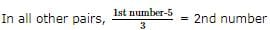Test: BITSAT Mock Test- 3 - Question 138

Complete the analogous pair.
Fog : Visibility : : AIDS : ?

Test: BITSAT Mock Test- 3 - Question 139

Group the following figures into three classes on the basis of identical properties.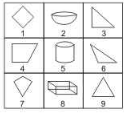Detailed Solution for Test: BITSAT Mock Test- 3 - Question 139

2,5,8 are all three-dimensional figures.
3,6,9 are all (two-dimensional) triangles.

Test: BITSAT Mock Test- 3 - Question 140

Find the missing character.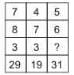Detailed Solution for Test: BITSAT Mock Test- 3 - Question 140

In the first column, 29 − 8 = 7 * 3 = 21.
In the second column, 19 − 7 = 4 * 3 = 12.
Let the missing number in the third column be x.
Then, 31 − 6 = 5 * x o r 5 x = 25 o r x = 5.

Test: BITSAT Mock Test- 3 - Question 141

In this letter series, some of the letters are missing. Choose the correct letter given below -
gfe _ ig _ eii _ fei _ gf _ ii

Test: BITSAT Mock Test- 3 - Question 142

In each question, three sequences of letters/numerals are given which correspond to each other. Find out the letters/numerals that come in vacant places.
C _ B _ D _ A _ B B D D
2 _ _ 4 _ 3 4 _ ? ? ? ?
_ a _ c b a _ d _ _ _ _

Test: BITSAT Mock Test- 3 - Question 143

Imagine your Information Technology business in a resort-like ambience. Your employees could have a holiday on every working day! Yes, God's own country is fast emerging as India's most happening Information Technology/Information Technology Enabled Services destination. The enviable tranquility, cleanliness, greenery and physical quality of life add to the aura. Consistently ranked as the No. 1 State in social achievements, Kerala is a dream destination for even the most discerning tourist. National Geographic Traveller has dubbed the State as "one of the ten paradises of the world". Not for nothing. Its natural beauty, picturesque backwaters, enchanting beaches, authentic ayurvedic rejuvenation centres and rich cultural heritage continue to hold people from all over the world, spellbound. But of late, the State is turning out to be a one-stop address for every Information Technology need as well.

Q.  Which one of the following statements is correct?

Test: BITSAT Mock Test- 3 - Question 144

Imagine your Information Technology business in a resort-like ambience. Your employees could have a holiday on every working day! Yes, God's own country is fast emerging as India's most happening Information Technology/Information Technology Enabled Services destination. The enviable tranquility, cleanliness, greenery and physical quality of life add to the aura. Consistently ranked as the No. 1 State in social achievements, Kerala is a dream destination for even the most discerning tourist. National Geographic Traveller has dubbed the State as "one of the ten paradises of the world". Not for nothing. Its natural beauty, picturesque backwaters, enchanting beaches, authentic ayurvedic rejuvenation centres and rich cultural heritage continue to hold people from all over the world, spellbound. But of late, the State is turning out to be a one-stop address for every Information Technology need as well.

Q. Which one of the following statements is correct?

Test: BITSAT Mock Test- 3 - Question 145

Imagine your Information Technology business in a resort-like ambience. Your employees could have a holiday on every working day! Yes, God's own country is fast emerging as India's most happening Information Technology/Information Technology Enabled Services destination. The enviable tranquility, cleanliness, greenery and physical quality of life add to the aura. Consistently ranked as the No. 1 State in social achievements, Kerala is a dream destination for even the most discerning tourist. National Geographic Traveller has dubbed the State as "one of the ten paradises of the world". Not for nothing. Its natural beauty, picturesque backwaters, enchanting beaches, authentic ayurvedic rejuvenation centres and rich cultural heritage continue to hold people from all over the world, spellbound. But of late, the State is turning out to be a one-stop address for every Information Technology need as well.

Q. Which one of the following statements is correct?

Test: BITSAT Mock Test- 3 - Question 146

Imagine your Information Technology business in a resort-like ambience. Your employees could have a holiday on every working day! Yes, God's own country is fast emerging as India's most happening Information Technology/Information Technology Enabled Services destination. The enviable tranquility, cleanliness, greenery and physical quality of life add to the aura. Consistently ranked as the No. 1 State in social achievements, Kerala is a dream destination for even the most discerning tourist. National Geographic Traveller has dubbed the State as "one of the ten paradises of the world". Not for nothing. Its natural beauty, picturesque backwaters, enchanting beaches, authentic ayurvedic rejuvenation centres and rich cultural heritage continue to hold people from all over the world, spellbound. But of late, the State is turning out to be a one-stop address for every Information Technology need as well.

Q. Which one of the following statements is correct?

Test: BITSAT Mock Test- 3 - Question 147

Select the correct mirror image of the figure (X) from amongst the given alternatives.

Q.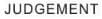Test: BITSAT Mock Test- 3 - Question 148

Select the correct mirror image of the figure (X) from amongst the given alternatives.

Q.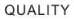Test: BITSAT Mock Test- 3 - Question 149

Each of the following questions consists of five Problem figures marked 1,2,3,4 and 5 followed by five Answer figures marked A,B,C,D and E . Select a figure from the Answer figures which will continue the same series as given in the Problem figures.

Q.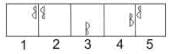Detailed Solution for Test: BITSAT Mock Test- 3 - Question 149

The symbol gets vertically inverted and laterally inverted alternately. It also moves in ACW direction through distances equal to two half-sides (of square boundary) and three half-sides alternately.

Test: BITSAT Mock Test- 3 - Question 150

Each of the following questions consists of five Problem figures marked 1,2,3,4 and 5 followed by five Answer figures marked A,B,C,D and E . Select a figure from the Answer figures which will continue the same series as given in the Problem figures.

Q.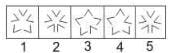## BITSAT Mock Tests Series & Past Year Papers

2 videos|15 docs|70 tests
 Use Code STAYHOME200 and get INR 200 additional OFF Use Coupon Code

## BITSAT Mock Tests Series & Past Year Papers

2 videos|15 docs|70 tests